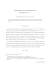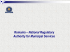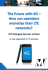# Approximation by Durrmeyer

## Transcription

Approximation by Durrmeyer
```ANNALES
POLONICI MATHEMATICI
LXIV.2 (1996)
Approximation by Durrmeyer-type operators
by Vijay Gupta and G. S. Srivastava (Roorkee)
Abstract. We define a new kind of Durrmeyer-type summation-integral operators and
study a global direct theorem for these operators in terms of the Ditzian–Totik modulus
of smoothness.
1. Durrmeyer  introduced modified Bernstein polynomials to approximate Lebesgue integrable functions on [0, 1], later motivated by the integral
modification of Bernstein polynomials by Durrmeyer; Sahai and Prasad 
and Mazhar and Totik  introduced modified Lupas operators and modified
Sz´
asz operators respectively to approximate Lebesgue integrable functions
on [0, ∞). A lot of work has been done on these three operators (see e.g.
, , – etc.). In a recent paper Heilmann  has studied the generalized operators which include all the three operators. We now give another
generalization of these operators as
(1.1)
Mn (f, x) =
∞
X
\
∞
pn,k (x)
bn,k (t)f (t) dt,
0
k=0
where
pn,k (x) = (−1)k
xk (k)
φ (x),
k! n
bn,k (t) = (−1)k+1
tk (k+1)
φ
(t)
k! n
and
(i)
(ii)
(iii)
φn (x) = (1 + cx)−n/c
−nx
φn (x) = e
φn (x) = (1 − x)n
for the interval [0, ∞) with c > 0,
for the interval [0, ∞) with c = 0,
for the interval [0, 1] with c = −1.
1991 Mathematics Subject Classification: 41A30, 41A36.
Key words and phrases: modulus of smoothness, global direct theorem, differential
and integral operators.
Research of the first author supported by Council of Scientific and Industrial Research,
India.

154
V. Gupta and G. S. Srivastava
The cases (i), (ii) and (iii) mentioned above give modified Baskakov
type operators, modified Sz´
asz-type operators and modified Bernstein type
polynomials respectively. The case (i) for c = 1 was recently introduced by
one of the authors (see e.g. ).
By Lr1 [0, ∞) we denote the class of functions g given by Lr1 [0, ∞) = {g :
g(r) ∈ L1 [0, a] for every a ∈ (0, ∞) and |g(r) (t)| ≤ M (1 + t)m , M and m are
constants depending on g}.
We remark that Lrp [0, ∞) is not contained in Lr1 [0, ∞) and L01 [0, ∞) =
L1 [0, ∞).
Following , the modulus of smoothness is given by
p
φ(x) = x(1 + cx),
ωφ2 (f, t)p = sup k∆2hφ f kp ,
0<h≤t
where
f (x − h) − 2f (x) + f (x + h) if [x − h, x + h] ⊆ [0, ∞),
0
otherwise.
This modulus of smoothness is equivalent to the modified K-functional (see
e.g. ) given by
∆φh f (x) =
n
K 2φ (f, t2 ) = inf{kf − gkp + t2 kφ2 g′′ kp + t4 kg′′ k : g ∈ W p2 (φ, [0, ∞))},
where
W p2 (φ, [0, ∞)) = {g ∈ Lp [0, ∞) : g′ ∈ ACloc [0, ∞), φ2 g′′ ∈ Lp [0, ∞)}.
In the present paper, we give a global direct theorem for the operators
(1.1) in terms of the Ditzian–Totik modulus of second order. Throughout
the paper, we denote by C positive constants not necessarily the same at
each occurrence.
2. In this section, we mention certain properties and results for the operators (1.1), which are necessary for the proof of the main result.
For the cases (i) and (ii), we have
∞
X
(2.1)
pn,k (x) = 1,
bn,k (t) = n,
k=0
k=0
∞
\
0
∞
X
1
pn,k (x) dx =
n−c
\
∞
and
bn,k (t) dt = 1,
0
and for the casep(iii) summation is from 0 to n and integration from 0 to 1.
For φ(x) = x(1 + cx), we have
(1)
(2.2)
φ2 (x)pn,k (x) = [k − nx]pn,k (x),
(1)
φ2 (t)bn,k (t) = [k − (n + c)t]bn,k (t).
Approximation by Durrmeyer-type operators
155
Lemma 1. For m, r ∈ N0 (the set of non-negative integers), if we define
Vr,n,m (x) =
∞
X
\
∞
pn+cr,k (x)
bn−cr,k+r (t)(t − x)m dt,
0
k=0
then
Vr,n,0 (x) = 1,
Vr,n,1 (x) =
(1 + r) + cx(1 + 2r)
n − c(r + 1)
and
Vr,n,2 (x)
=
2cx2 (n + 2cr 2 + 4cr + c) + 2x(n + 2cr 2 + 5cr + 2c) + r 2 + 3r + 2
.
[n − c(r + 1)][n − c(r + 2)]
Further , we have the recurrence relation
(1)
[n − c(m + r + 1)]Vr,n,m+1 (x) = φ2 (x)[Vr,n,m
(x) + 2mVr,n,m−1 (x)]
+ [(1 + 2cx)(m + r + 1) − cx]Vr,n,m (x).
By using (2.1) and (2.2) the proof of the above lemma easily follows
along the lines of  and .
It may be remarked that for all x ∈ [0, ∞) (cases (i) and (ii)) and for all
x ∈ [0, 1] (case (iii)), we have
Vr,n,m (x) = O(n−[(m+1)/2] ).
Lemma 2. If f ∈ Lrp [0, ∞) ∪ Lr1 [0, ∞), 1 < p ≤ ∞ and x ∈ [0, ∞), we
have
∞
∞
\
X
pn+cr,k (x) bn−cr,k+r (t)f (r) (t) dt,
(2.3)
Mn(r) (f, x) = α(n, r, c)
0
k=0
where
α(n, r, c) =
r−1
Y
l=0
n + cl
.
n − c(l + 1)
We see that the operators defined by (2.3) are not positive. To make the
operators positive, we introduce the operators
Mn,r f ≡ D r Mn I r f,
f ∈ Lp [0, ∞) ∪ L1 [0, ∞),
where D and I are the differential and integral operators respectively.
Therefore, we define the operators by
Mn,r (f, x) ≡ α(n, r, c)
∞
X
k=0
\
∞
pn+cr,k (x)
bn−cr,k+r (t)f (t) dt,
0
f ∈ Lp [0, ∞) ∪ L1 [0, ∞), n > (c + m)r.
156
V. Gupta and G. S. Srivastava
(r)
The operators Mn,r are positive and the quantity kMn f − f (r) kp , f ∈
Lrp [0, ∞), is equivalent to kMn,r f − f kp , f ∈ Lp [0, ∞).
Using (2.1), we can easily prove that for n > c(r +1), kMn,r f k1 ≤ Ckf k1
for f ∈ L1 [0, ∞) and kMn,r f k∞ ≤ Ckf k∞ for f ∈ L∞ [0, ∞). Making use of
the Riesz–Thorin theorem, we get
(2.4)
kMn,r f kp ≤ Ckf kp ,
f ∈ Lp [0, ∞), 1 ≤ p ≤ ∞, n > c(r + 1).
Corollary 3. For every m ∈ N0 , n > c(r + 2m + 1) and x ∈ [0, ∞), we
have
(2.5)
|Mn,r ((t − x)2m , x)| ≤ Cn−m (φ2 (x) + n−1 )m ,
|Mn,r ((t − x)2m+1 , x)| ≤ C(1 + 2x)n−m−1 (φ2 (x) + n−1 )m ,
where the constant C is independent of n. For fixed x ∈ [0, ∞), we obtain
(2.6)
|Mn,r ((t − x)m , x)| = O(n−[(m+1)/2] ),
n → ∞.
P r o o f. Since Mn,r ((t − x)m , x) = α(n, r, c)Vr,n,m (x) the estimate (2.5)
follow from (2.2) along the lines of ; (2.6) immediately follows from (2.5).
Lemma 4. Let t ∈ [0, ∞) and n > c(r + m). Then
Mn,r ((1 + t)−m , x) ≤ C(1 + cx)−m ,
x ∈ [0, ∞),
where the constant C is independent of n.
P r o o f. It is easily verified that
(1 + ct)−m bn−cr,k+r (t) =
m−1
Y
l=0
n − cr + lc
bn−cr+mc,k+r (t)
n + lc + kc + 1
and
pn+cr,k (x) = (1 + cx)−m
m
Y
n + cr − lc + kc
l=0
n + cr − lc
pn+cr−mc,k (x).
Making use of these two identities and (2.1), we get
Mn,r ((1 + t)−m , x)
∞
∞
\
X
pn+cr,k (x) bn−cr,k+r (t)(1 + t)−m dt
= α(n, r, c)
= α(n, r, c)
k=0
0
∞
X
m−1
Y
k=0
pn+cr,k (x)
l=0
n − cr + lc
n + lc + kc + 1
\
∞
bn−cr+mc,k+r (t) dt
0
Approximation by Durrmeyer-type operators
= α(n, r, c)
∞
X
157
(1 + cx)−m pn+cr−mc,k (x)
k=0
×
m
m−1
Y
n + cr − lc + kc Y
l=1
n + cr − lc
≤ C(1 + cx)−m
∞
X
l=0
n − cr + lc
n + lc + kc + 1
pn+cr−mc,k (x) = C(1 + cx)−m .
k=0
For the two monomials e0 , e1 and x ∈ [0, ∞), n → ∞, we obtain by
direct computation
(2.7)
Mn,r (e0 , x) = 1 + O(n−1 ),
(2.8)
Mn,r (e1 , x) = x(1 + O(n−1 )).
Lemma 5. For
∞
n∞
\u\ u\∞\o X
Hn (u) =
pn+cr,k (x)bn−cr,k+r (t)(u − t) dt dx,
−
0 0
we have Hn (u) ≤ Cn
0 0
k=0
−1 2
φ (u), where C is independent of n and u.
The proof easily follows by using (2.1) along the lines of [1, Lemma 5.2].
3. In this section, we prove the following global direct theorem.
Theorem 1. Suppose f ∈ Lp [0, ∞), 1 ≤ p < ∞, n > c(r + 5). Then
kMn,r f − f kp ≤ C{ωφ2 (f, n−1/2 ) + n−1 kf kp },
where the constant C is independent of n.
P r o o f. By Taylor’s expansion of g, we have
(3.1)
t
\
g(t) = g(x) + (t − x)g′ (x) + (t − u)g′′ (u) du.
x
Next, since Mn,r (f, x) are uniformly bounded operators, for every g ∈
W p2 (φ, [0, ∞)), we have
(3.2)
kMn,r f − f kp ≤ Ckf − gkp + kMn,r g − gkp .
Using (2.5), (2.8) and (3.1) and following , we have
(3.3) kMn,r g − gkp ≤ C{kgkp + kg′ kLp [0,1] }
+ k(1 + 2crx)g′ kLp [0,∞) + kMn,r (R(g, t, x), ·)kp
where R(g, t, x) =
Tt
x
≤ Cn−1 [kgkp + kφ2 g′′ kp ] + kMn,r (R(g, t, x), x)kp ,
g′′ (u)(t − u) du.
158
V. Gupta and G. S. Srivastava
Now, we prove that
(3.4)
kMn,r (R(g, t, x), x)kp ≤ Cn−1 k(φ2 + n−1 )g′′ kp .
We prove this for p = 1 and p = ∞. The case 1 < p < ∞ follows again by
the Riesz–Thorin theorem.
Using (2.5) for the case m = 1 and Lemma 4, the case p = ∞ easily
follows (see e.g. ).
For p = 1, we derive (3.4) by applying Fubini’s theorem twice, the definition of Hn (u) and Lemma 5:
\
∞
|Mn,r (R(g, t, x), x)| dx
0
≤ α(n, r, c)
\
∞X
∞
pn+cr,k (x)
0 k=0
\
∞
= α(n, r, c)
×
∞
X
0
t
\
′′
bn−cr,k+r (t) g (u)(t − u) du dt dx
\\ \\
n∞u
|g′′ (u)|
0
\
∞
0 0
−
u∞o
x
(u − t)
0 0
pn+cr,k (x)bn−cr,k+r (t) dt dx du
k=0
\
∞
= α(n, r, c)
|g′′ (u)|Hn (u) du
0
≤ Cn
−1
2 ′′
kφ g k1 ≤ Cn−1 k(φ2 + n−1 )g′′ k1 ,
where C is independent of n. Hence (3.4) holds by the Riesz–Thorin theorem
for 1 ≤ p < ∞. Combining the estimates (3.2), (3.3) and (3.4), we get
kMn,r f − f kp = Ckf − gkp + Cn−1 {kf − gkp + kf kp
+ kφ2 g′′ kp + k(φ2 + n−1 )g′′ kp }
≤ C{kf − gkp + n−1 kφ2 g′′ kp + n−2 kg′′ kp + n−1 kf kp }.
Next taking the infimum over all g ∈ W 2p (φ, [0, ∞)) on the right hand side,
we get
kMn,r f − f kp ≤ C{K 2φ (f, n−1 ) + n−1 kf kp }.
This completes the proof of Theorem 1.
R e m a r k. The conclusion of the above theorem is true for the space
Lp [0, ∞), 1 ≤ p < ∞ (i.e. limn→∞ kMn,r f − f kp = 0 for every f ∈
Lp [0, ∞)) since the basic fact about the Ditzian–Totik modulus of smooth-
Approximation by Durrmeyer-type operators
159
ness ωφ2 (f, n−1 ) is that
lim ωφ2 (f, n−1 ) = 0
n→∞
for all f ∈ Lp [0, ∞) if 1 ≤ p < ∞,
or for all bounded functions f ∈ C[0, ∞) which satisfy
lim f (x) = L∞ < ∞
x→∞
if p = ∞ (cf. [3, p. 36]).
References










M. M. D e r r i e n n i c, Sur l’approximation de fonctions int´egrables sur [0, 1] par des
polynˆ
omes de Bernstein modifi´es, J. Approx. Theory 31 (1981), 325–343.
Z. D i t z i a n and K. I v a n o v, Bernstein type operators and their derivatives, ibid.
56 (1989), 72–90.
Z. D i t z i a n and V. T o t i k, Moduli of Smoothness, Springer Ser. Comput. Math. 9,
Springer, Berlin, 1987.
J. L. D u r r m e y e r, Une formule d’inversion de la transform´ee de Laplace: Applications `
a la th´eorie des moments, Th`ese de 3e Cycle, Facult´e des Sciences de
l’Universit´e de Paris, 1967.
V. G u p t a, A note on modified Baskakov type operators, Approx. Theory Appl. 10
(1994), 74–78.
M. H e i l m a n n, Direct and converse results for operators of Baskakov–Durrmeyer
type, ibid. 5 (1989), 105–127.
H. S. K a s a n a, P. N. A g r a w a l and V. G u p t a, Inverse and saturation theorems
for linear combination of modified Baskakov operators, ibid. 7 (1991), 65–82.
S. M. M a z h a r and V. T o t i k, Approximation by modified Sz´
asz operators, Acta
Sci. Math. (Szeged) 49 (1985), 257–269.
A. S a h a i and G. P r a s a d, On simultaneous approximation by modified Lupas operators, J. Approx. Theory 45 (1985), 122–128.
R. P. S i n h a, P. N. A g r a w a l and V. G u p t a, On simultaneous approximation by
modified Baskakov operators, Bull. Soc. Math. Belg. S´er. B 42 (1991), 217–231.
Department of Mathematics
University of Roorkee
Roorkee 247 667, India
E-mail: maths%[email protected]
Current address of Vijay Gupta:
Department of Mathematics
Institute of Engineering and Technology
Rohilkhand University
Bareilly 243006, India
Re¸cu par la R´edaction le 27.10.1994
R´evis´e le 27.7.1995
```

### DAILY PLANT INSPECTION CHECKLIST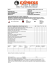### HOW TO GET STARTED AS AN AMRRON OPERATOR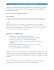### Corporate Factsheet_English### Cat Link Technology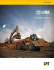### North Atlantic MNPS Airspace Map### Certificate IV In Warehousing Operations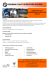### Tilgin launches the HG2380, a true high-end Home Gateway PRESS RELEASE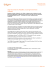### The Essential Spectra of Toeplitz Operators with Symbols in Hâ + C